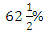# If x is 80% of y, then what percent of 2x is y

1.  40%

2.

3.

4.  80%

4Explanation :
No Explanation available for this question

# Subtracting 6% of x from x is equivalent to multiplying x by how much

1.  0.094

2.  0.94

3.  9.4

4.  94

4

0.94

Explanation :
No Explanation available for this question

# (x% of y + y% of x )=

1.  x% of y

2.  y% of x

3.  2% of xy

4.  Xy% of 3

4

2\% of xy

Explanation :
No Explanation available for this question

# If A is 150 percent of B, then B is what percent of (A + B)

1.

2.  40%

3.

4.  75%

4

40\%

Explanation :
No Explanation available for this question

# If 8% of x = 4% of y, then 20% of x is :

1.  10% of y

2.  16% of y

3.  80% of y

4.  None of these

4

10\% of y

Explanation :
No Explanation available for this question

# If 20% of A = B and 40% of B = C , then 60% of (A + B) is:

1.  30% of C

2.  60% of C

3.  75% of C

4.  None of these

4

None of these

Explanation :
No Explanation available for this question

# If x% of a is the same as y% of b, then z% of b is :

1.  xy/z % of a

2.  yz/x % of a

3.  xz/y % of a

4.  none of these

4

xz/y \% of a

Explanation :
No Explanation available for this question

# Which of the following statement(s) reflects India's stand on new World Trade Organization's proposal on the issue of the subsidy to agro products (1) India would protect the interest of its poor farmers who can not bear the burden of the cost of the products if they have to sell them on cheaper rate. (2) Indian industry needs more flexibility as compared to the industry in developed countries. (3) WTO has set up a new committee under the chairmanship of Putin of Russia to look into the issue of subsidy and find out a solution to the problem acceptable to all the parties.

1.  Only 1

2.  Only 2

3.  Only 3

4.  Both 1 & 2

5.  None of these

5

Only 1

Explanation :
No Explanation available for this question

# If A = x% of y and B = y% of x, then which of the following is true

1.  A is smaller than B

2.  A is greater than B.

3.  Relationship between A and B cannot be determined

4.  If x is smaller than y, then A is greater than B.

5.  None of these

5

None of these

Explanation :
No Explanation available for this question

1.  500

2.  1000

3.  2000

4.  2250

4